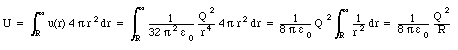## Solutions Homework Set # 4 - Physics 122

Problem 1

The electrostatic potential due to a point charge q is equal to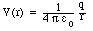Based on this equation we can conclude that:

• The potential close to a negatively charged object becomes increasingly negative.
• The potential close to a positively charged object becomes increasingly positive.
• The potential close to a charged object is proportional to the charge of the object.

The electric field E and the potential V are related as follows: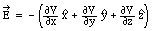Based on this equation we conclude that the larger the difference in the electrostatic potential over a certain distance, the larger the magnitude of the electric field at that position.

Problem 2

Using the contour plot you can determined the potential difference [Delta]V between the initial and final position of charge q (make sure you get the sign correct). The initial and final potential energy of the charge is (q V1) and (q V2), respectively. The work required to move the charge q is thus equal to -[Delta]U = -(q V2 - q V1) = -q [Delta]V.

Problem 3

The electric field is directed perpendicular to the equipotential contours. Using the ruler in Figure you can determine the change the potential per unit distance:Make sure you use the correct units for both [Delta]V and [Delta]d.

Problem 4

Use the same technique used in Problem 3 to calculate the magnitude of the electric field E. The magnitude of the force on a charge q will then be q E.

Problem 5

Pick a certain point on the equipotential surface closest to the charge of interest. The value of the potential at that point is equal to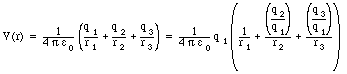Even though the other two charges are further away, they will still have a significant influence on the potential due to the 1/r dependence of the potential. Use the given ratio of the charges and the measured distances to determine the unknown charge.

Problem 6

Consider a sphere of radius R with a total charge Q. The electric field outside the sphere is that of a point charge Q located at the center of the sphere: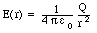The value of the electric field on the surface of the sphere is thus equal to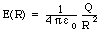In the same manner we can determine the electrostatic potential on the surface of the sphere: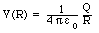Combining the last two equations we obtain: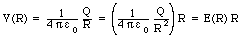Problem 7

Using the expression for V(R) we can determine the charge on the dome: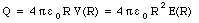Problem 8

Consider a spherical shell of inner radius ri and outer radius ro. Assuming that the total charge on the shell is Q, the charge density of the shell is equal to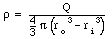The electric field generated by the charge distribution will have spherical symmetry and can be easily obtained using Gauss' law: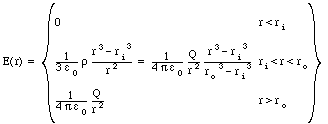To obtain the electrostatic potential at a certain point P, located a distance r from the center of the spherical shell, we have to integrate the electric field over a path from infinity to this point P. Consider three special cases:

• at r > ro: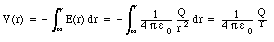• at ro > r > ri: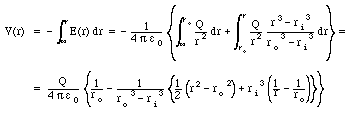• at ri > r: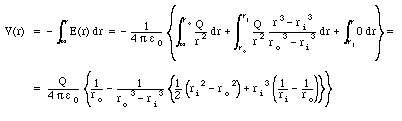The potential at the center of the spherical shell is thus equal to: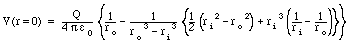Problem 9

Since the electric field inside the spherical shell is equal to 0 N/C, the potential is constant inside the spherical shell. The potential on the inside of the shell will thus be equal to the potential at the center. Thus: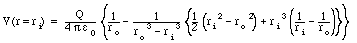Problem 10

The potential on the outer surface of the shell can be obtained from the general solution discussed in Problem 8: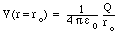Problem 11

The electrostatic potential outside a charged sphere, of radius R and total charge Q, is the same as the electrostatic potential generated by a point charge Q located at the center of the sphere: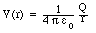The electrostatic potential on the surface of the sphere is thus equal toThis equation can be used to express the charge Q in terms of the potential on the sphere and the radius R: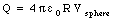Problem 12

The electric field inside the metal sphere is zero. In order to determine the total energy associated with the electric field we thus have to integrate the energy density over the entire volume outside the sphere. The electric field outside a charged sphere, of radius R and total charge Q, is the same as the electric field generated by a point charge Q located at the center of the sphere:The energy density u(r) is thus equal to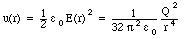The total energy associated with the electric field is thus equal to: